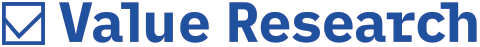# Bear Hug

##### A long-term investor tries to time valuation rather than price. Such an investor will buy when equity is priced below or around fair-value

Long-term investors should welcome bear markets. By definition, a bear market creates depressed prices over a long time period. This gives the long-term investor the chance to generate a larger corpus of savings and invest those savings at low valuations.

A long-term investor tries to time valuation rather than price; he will buy when equity is priced below or at around fair-value. There are, however, different methods of judging fair-value and these are all, to an extent, subjective. One common method involves examining price-earnings ratios (PE) and earnings (EPS) growth rates. This is the PEG or Price Earnings Growth ratio. The PEG is derived by dividing PE by an estimated EPS growth rate. Stocks are fair-value when PEG =1 or less.

Another method involves comparing the PE ratio to a discount rate of return (FD rates, for instance) by inverting it to derive earnings over price. A PE of 20 equals an "EP" of 5 per cent. If the discount rate is over 5 per cent, the equity market is over-valued by this method.

These two methods can throw up contradictory results. In a high-growth scenario, the market is often over-valued in terms of discount rate but under-valued in terms of PEG.

For example, assume EPS growth at 30 per cent and a market-wide PE of 20 with the discount rate at 8 per cent. The PEG is 0.67 - very reasonable. But the “EP” of 5 is below investment-grade. The discount rate implies PE of 12-13 is fair-value. This was almost exactly the scenario in the Nifty, about a year ago.

In a low-interest scenario, a high PE may be justified by a low discount rate but the PEG could be inflated. For example, Japanese rates stayed near 0 per cent through a decade (1994-2004) so triple-digit PEs could be justified by low discount rates. However EPS growth was about 1 per cent for the Nikkei-225 so PEGs were absurdly high.

The ideal situation is naturally, one where both valuation metrics offer "buy" signals. That actually happened through much of the 2003-2007 bull market.

Between 2002-3 and 2006-7, EPS growth for the Nifty averaged over 25 per cent per annum - a PEG analysis would suggest that PE ratios of up to 25 were justifiable. If we use the yield to maturity of the 364-day T-Bill as our discount rate, the YTM stayed below 5 per cent until late 2004 and below 7 per cent until June 2006 implying that PE ratios of up to 20 were justifiable through this period.

Actually Nifty PE ratios stayed well below 20 until May 2006. By late 2005, the discount rate comparison suggested that valuations were stretched but PEGs were below 1. By April 2007, PEG ratios also suggested that the valuations were stretched.

What would be comfortable valuation levels in the context of 2007-8 estimates and Q1 results? The guesstimate on the basis of Q1 results is that EPS growth for the Nifty will slow down to about 15 per cent in 2007-8. Hence, by the PEG, the fair-value PE ratio for the Nifty would have to be capped at about 15. On the basis of estimated 2007-8 earnings, fair-value is at about Nifty 3700.

The 364-D T-Bill has eased down a little from nearly 8 per cent to just about 7.5 per cent in the latest auction. Most analysts assume that rupee interest rates have more or less stabilised. Let's assume the YTM will wander through 7-8 per cent in this fiscal. That suggests PEs of 12-14 are close to fair valuations. Again, using our estimate of 15 per cent growth in 2007-8 EPS, that works out to a Nifty of 3500 or less. Hence, despite the correction from 4600-levels, a long-term investor should still be inclined to wait, rather than buy across the board at current levels. There are several ways in which the situation might change. One is a deeper correction till 3500 levels - then both measures offer buy signals. A second possibility is lower interest rates - current valuations would be justified if the YTM dropped to about 5 per cent even though PEG would stay high. The third possibility is a sharp acceleration in EPS growth in the second half of 2007-8. An EPS growth rate of 25-30 per cent would pull the PEG below 1 though the discount rate would still suggest a sell.

Our long-term investor would be accumulating a corpus of savings, parking it in FDs and waiting for any of these three events to offer a safer entry-point.

(Nifty PE valuations have been taken from NSE and T-Bill YTM rates from RBI)